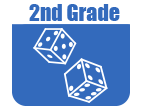Resources by Strand

Strand: MATHEMATICAL PRACTICES (2.MP)
The Standards for Mathematical Practice in Second Grade describe mathematical habits of mind that teachers should seek to develop in their students. Students become mathematically proficient in engaging with mathematical content and concepts as they learn, experience, and apply these skills and attitudes (Standards 2.MP.1-6).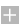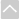Strand: OPERATIONS AND ALGEBRAIC THINKING (2.OA)
Represent and solve problems involving addition and subtraction (Standards 2.OA.1)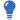Core Guide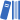OER Curriculum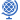Instructional Tasks

Standard 2.OA.1
Use addition and subtraction within 100 to solve one- and two-step word problems involving situations of adding to, taking from, putting together, taking apart, and comparing with unknowns in all positions, for example, by using drawings and equations with a symbol for the unknown number to represent the problem.

Fluently add and subtract within 20 (Standards 2.OA.2).Core GuideOER CurriculumInstructional TasksReview Activitiy

Standard 2.OA.2
Fluently add and subtract within 20.

1. Add and subtract within 20 using mental strategies such as counting on; making ten (for example, 8 + 6 = 8 + 2 + 4 = 10 + 4 = 14); decomposing a number leading to a ten (for example, 13 - 4 = 13 - 3 - 1 = 10 - 1 = 9); using the relationship between addition and subtraction (for example, knowing that 8 + 4 = 12, one knows 12 - 8 = 4); and creating equivalent but easier or known sums (for example, adding 6 + 7 by creating the known equivalent 6 + 6 + 1 = 12 + 1 = 13).
2. By the end of Grade 2, know from memory all sums of two one-digit numbers.
Work with equal groups of objects to gain foundations for multiplication (Standards 2.OA.3–4).Core GuideOER CurriculumInstructional TasksReview Activitiy

Standard 2.OA.3
Determine whether a group of objects (up to 20) has an odd or even number of members, (for example, by pairing objects or counting them by twos). Write an equation to express an even number as a sum of two equal addends.

Standard 2.OA.4
Use addition to find the total number of objects arranged in rectangular arrays with up to 5 rows and up to 5 columns; write an equation to express the total as a sum of equal addends.Strand: NUMBER AND OPERATIONS IN BASE TEN (2.NBT)
Understand place value (Standards 2.NBT.1–4).Core GuideOER CurriculumInstructional TasksReview Activitiy

Standard 2.NBT.1
Understand that the three digits of a three-digit number represent amounts of hundreds, tens, and ones; for example, 706 equals 7 hundreds, 0 tens, and 6 ones. Understand the following as special cases:

1. 100 can be thought of as a bundle of ten tens called a "hundred."
2. The numbers 100, 200, 300, 400, 500, 600, 700, 800, 900 refer to one, two, three, four, five, six, seven, eight, or nine hundreds (and 0 tens and 0 ones).

Standard 2.NBT.2
Count within 1,000; skip-count by fives, tens, and hundreds.

Standard 2.NBT.3
Read and write numbers to 1,000 using base-ten numerals, number names, and expanded form.

Standard 2.NBT.4
Compare two three-digit numbers based on meanings of the hundreds, tens, and ones digits, using >, =, and < symbols to record the results of comparisons.

They use place value understanding and properties of operations to add and subtract (Standards 2.NBT.5–9).Core GuideOER CurriculumInstructional TasksReview Activitiy

Standard 2.NBT.5
Fluently add and subtract within 100 using strategies based on place value, properties of operations, and/or the relationship between addition and subtraction.

Standard 2.NBT.6
Add up to four two-digit numbers using strategies based on place value and properties of operations.

Standard 2.NBT.7
Add and subtract within 1,000 using concrete models or drawings and strategies based on place value, properties of operations, and/or the relationship between addition and subtraction; relate the strategy to a written method. Understand that in adding or subtracting three-digit numbers, one adds or subtracts hundreds and hundreds, tens and tens, and ones and ones, and that it is sometimes necessary to compose or decompose tens or hundreds.

Standard 2.NBT.8
Mentally add 10 or 100 to a given number 100-900, and mentally subtract 10 or 100 from a given number 100-900.

Standard 2.NBT.9
Explain why addition and subtraction strategies work, using place value and the properties of operations. Explanations may be supported by drawings or objectsStrand: MEASUREMENT AND DATA (2.MD)
Measure and estimate lengths in standard units (Standards 2.MD.1–4).Core GuideOER CurriculumInstructional Tasks

Standard 2.MD.1
Measure the length of an object by selecting and using appropriate tools such as rulers, yardsticks, meter sticks, and measuring tapes.

Standard 2.MD.2
Measure the length of an object twice, using length units of different lengths for the two measurements; describe how the two measurements relate to the size of the unit chosen.

Standard 2.MD.3
Estimate lengths using units of inches, feet, centimeters, and meters.

Standard 2.MD.4
Measure to determine how much longer one object is than another, expressing the length difference in terms of a standard length unit. For example, after measuring a pencil and a crayon, a student uses the measurements to determine that the pencil is two inches longer than the crayon.

Relate addition and subtraction to length (Standards 2.MD.5–6).Core GuideOER CurriculumInstructional Tasks

Standard 2.MD.5
Use addition and subtraction within 100 to solve word problems involving lengths that are given in the same units. For example, use drawings (such as drawings of rulers) and equations with a symbol for the unknown number to represent the problem.

Standard 2.MD.6
Represent whole numbers as lengths from 0 on a number line diagram with equally spaced points corresponding to the numbers 0, 1, 2... Represent whole number sums and differences within 100 on a number line diagram.

They work with time and money (Standards 2.MD.7–8).Core GuideOER CurriculumInstructional TasksReview Activitiy

Standard 2.MD.7
Tell and write time from analog and digital clocks to the nearest five minutes, using a.m. and p.m.

Standard 2.MD.8
Solve word problems involving dollar bills, quarters, dimes, nickels, and pennies, using \$ and ¢ symbols appropriately. For example, if you have 2 dimes and 3 pennies, how many cents do you have?

They represent and interpret data (Standards 2.MD.9–10).Core GuideOER CurriculumInstructional TasksReview Activitiy

Standard 2.MD.9
Generate measurement data by measuring lengths of several objects to the nearest whole unit, or by making repeated measurements of the same object. Show the measurements by making a line plot, where the horizontal scale is marked off in whole-number units.

Standard 2.MD.10
Draw a picture graph and a bar graph (with single-unit scale) to represent a data set with up to four categories. Solve simple put-together, take-apart, and comparison problems using information presented in a bar graph.Strand: GEOMETRY (2.G)
Reason with shapes and their attributes. (Standards 2.G.1–3).Core GuideOER CurriculumInstructional TasksReview Activitiy

Standard 2.G.1
Recognize and draw shapes having specified attributes, such as a given number of angles or a given number of equal faces. Sizes are compared directly or visually, not compared by measuring. Identify triangles, quadrilaterals, pentagons, hexagons, and cubes.

Standard 2.G.2
Partition a rectangle into rows and columns of same-size squares and count to find the total number of squares.

Standard 2.G.3
Partition circles and rectangles into two, three, or four equal shares; describe the shares using the words halves, thirds, half of, a third of, etc.; and describe the whole as two halves, three thirds, or four fourths. Recognize that equal shares of identical wholes need not have the same shape.The Online Core Resource pages are a collaborative project between the Utah State Board of Education and the Utah Education Network. If you would like to recommend a high quality resource, contact Trish French (Elementary) or Lindsey Henderson (Secondary). If you find inaccuracies or broken links contact resources@uen.org.